# sklearn.model_selection.GridSearchCV¶

class sklearn.model_selection.GridSearchCV(estimator, param_grid, *, scoring=None, n_jobs=None, refit=True, cv=None, verbose=0, pre_dispatch='2*n_jobs', error_score=nan, return_train_score=False)[source]

Exhaustive search over specified parameter values for an estimator.

Important members are fit, predict.

GridSearchCV implements a “fit” and a “score” method. It also implements “score_samples”, “predict”, “predict_proba”, “decision_function”, “transform” and “inverse_transform” if they are implemented in the estimator used.

The parameters of the estimator used to apply these methods are optimized by cross-validated grid-search over a parameter grid.

Read more in the User Guide.

Parameters:
estimatorestimator object

This is assumed to implement the scikit-learn estimator interface. Either estimator needs to provide a score function, or scoring must be passed.

param_griddict or list of dictionaries

Dictionary with parameters names (str) as keys and lists of parameter settings to try as values, or a list of such dictionaries, in which case the grids spanned by each dictionary in the list are explored. This enables searching over any sequence of parameter settings.

scoringstr, callable, list, tuple or dict, default=None

Strategy to evaluate the performance of the cross-validated model on the test set.

If scoring represents a single score, one can use:

If scoring represents multiple scores, one can use:

• a list or tuple of unique strings;

• a callable returning a dictionary where the keys are the metric names and the values are the metric scores;

• a dictionary with metric names as keys and callables a values.

See Specifying multiple metrics for evaluation for an example.

n_jobsint, default=None

Number of jobs to run in parallel. None means 1 unless in a joblib.parallel_backend context. -1 means using all processors. See Glossary for more details.

Changed in version v0.20: n_jobs default changed from 1 to None

refitbool, str, or callable, default=True

Refit an estimator using the best found parameters on the whole dataset.

For multiple metric evaluation, this needs to be a str denoting the scorer that would be used to find the best parameters for refitting the estimator at the end.

Where there are considerations other than maximum score in choosing a best estimator, refit can be set to a function which returns the selected best_index_ given cv_results_. In that case, the best_estimator_ and best_params_ will be set according to the returned best_index_ while the best_score_ attribute will not be available.

The refitted estimator is made available at the best_estimator_ attribute and permits using predict directly on this GridSearchCV instance.

Also for multiple metric evaluation, the attributes best_index_, best_score_ and best_params_ will only be available if refit is set and all of them will be determined w.r.t this specific scorer.

See scoring parameter to know more about multiple metric evaluation.

See Custom refit strategy of a grid search with cross-validation to see how to design a custom selection strategy using a callable via refit.

Changed in version 0.20: Support for callable added.

cvint, cross-validation generator or an iterable, default=None

Determines the cross-validation splitting strategy. Possible inputs for cv are:

• None, to use the default 5-fold cross validation,

• integer, to specify the number of folds in a (Stratified)KFold,

• An iterable yielding (train, test) splits as arrays of indices.

For integer/None inputs, if the estimator is a classifier and y is either binary or multiclass, StratifiedKFold is used. In all other cases, KFold is used. These splitters are instantiated with shuffle=False so the splits will be the same across calls.

Refer User Guide for the various cross-validation strategies that can be used here.

Changed in version 0.22: cv default value if None changed from 3-fold to 5-fold.

verboseint

Controls the verbosity: the higher, the more messages.

• >1 : the computation time for each fold and parameter candidate is displayed;

• >2 : the score is also displayed;

• >3 : the fold and candidate parameter indexes are also displayed together with the starting time of the computation.

pre_dispatchint, or str, default=’2*n_jobs’

Controls the number of jobs that get dispatched during parallel execution. Reducing this number can be useful to avoid an explosion of memory consumption when more jobs get dispatched than CPUs can process. This parameter can be:

• None, in which case all the jobs are immediately created and spawned. Use this for lightweight and fast-running jobs, to avoid delays due to on-demand spawning of the jobs

• An int, giving the exact number of total jobs that are spawned

• A str, giving an expression as a function of n_jobs, as in ‘2*n_jobs’

error_score‘raise’ or numeric, default=np.nan

Value to assign to the score if an error occurs in estimator fitting. If set to ‘raise’, the error is raised. If a numeric value is given, FitFailedWarning is raised. This parameter does not affect the refit step, which will always raise the error.

return_train_scorebool, default=False

If False, the cv_results_ attribute will not include training scores. Computing training scores is used to get insights on how different parameter settings impact the overfitting/underfitting trade-off. However computing the scores on the training set can be computationally expensive and is not strictly required to select the parameters that yield the best generalization performance.

New in version 0.19.

Changed in version 0.21: Default value was changed from True to False

Attributes:

A dict with keys as column headers and values as columns, that can be imported into a pandas DataFrame.

For instance the below given table

param_kernel

param_gamma

param_degree

split0_test_score

rank_t…

‘poly’

2

0.80

2

‘poly’

3

0.70

4

‘rbf’

0.1

0.80

3

‘rbf’

0.2

0.93

1

will be represented by a cv_results_ dict of:

{
'param_kernel': masked_array(data = ['poly', 'poly', 'rbf', 'rbf'],
mask = [False False False False]...)
'param_gamma': masked_array(data = [-- -- 0.1 0.2],
mask = [ True  True False False]...),
'param_degree': masked_array(data = [2.0 3.0 -- --],
mask = [False False  True  True]...),
'split0_test_score'  : [0.80, 0.70, 0.80, 0.93],
'split1_test_score'  : [0.82, 0.50, 0.70, 0.78],
'mean_test_score'    : [0.81, 0.60, 0.75, 0.85],
'std_test_score'     : [0.01, 0.10, 0.05, 0.08],
'rank_test_score'    : [2, 4, 3, 1],
'split0_train_score' : [0.80, 0.92, 0.70, 0.93],
'split1_train_score' : [0.82, 0.55, 0.70, 0.87],
'mean_train_score'   : [0.81, 0.74, 0.70, 0.90],
'std_train_score'    : [0.01, 0.19, 0.00, 0.03],
'mean_fit_time'      : [0.73, 0.63, 0.43, 0.49],
'std_fit_time'       : [0.01, 0.02, 0.01, 0.01],
'mean_score_time'    : [0.01, 0.06, 0.04, 0.04],
'std_score_time'     : [0.00, 0.00, 0.00, 0.01],
'params'             : [{'kernel': 'poly', 'degree': 2}, ...],
}


NOTE

The key 'params' is used to store a list of parameter settings dicts for all the parameter candidates.

The mean_fit_time, std_fit_time, mean_score_time and std_score_time are all in seconds.

For multi-metric evaluation, the scores for all the scorers are available in the cv_results_ dict at the keys ending with that scorer’s name ('_<scorer_name>') instead of '_score' shown above. (‘split0_test_precision’, ‘mean_train_precision’ etc.)

best_estimator_estimator

Estimator that was chosen by the search, i.e. estimator which gave highest score (or smallest loss if specified) on the left out data. Not available if refit=False.

See refit parameter for more information on allowed values.

best_score_float

Mean cross-validated score of the best_estimator

For multi-metric evaluation, this is present only if refit is specified.

This attribute is not available if refit is a function.

best_params_dict

Parameter setting that gave the best results on the hold out data.

For multi-metric evaluation, this is present only if refit is specified.

best_index_int

The index (of the cv_results_ arrays) which corresponds to the best candidate parameter setting.

The dict at search.cv_results_['params'][search.best_index_] gives the parameter setting for the best model, that gives the highest mean score (search.best_score_).

For multi-metric evaluation, this is present only if refit is specified.

scorer_function or a dict

Scorer function used on the held out data to choose the best parameters for the model.

For multi-metric evaluation, this attribute holds the validated scoring dict which maps the scorer key to the scorer callable.

n_splits_int

The number of cross-validation splits (folds/iterations).

refit_time_float

Seconds used for refitting the best model on the whole dataset.

This is present only if refit is not False.

New in version 0.20.

multimetric_bool

Whether or not the scorers compute several metrics.

classes_ndarray of shape (n_classes,)

Class labels.

n_features_in_int

Number of features seen during fit.

feature_names_in_ndarray of shape (n_features_in_,)

Names of features seen during fit. Only defined if best_estimator_ is defined (see the documentation for the refit parameter for more details) and that best_estimator_ exposes feature_names_in_ when fit.

New in version 1.0.

ParameterGrid

Generates all the combinations of a hyperparameter grid.

train_test_split

Utility function to split the data into a development set usable for fitting a GridSearchCV instance and an evaluation set for its final evaluation.

sklearn.metrics.make_scorer

Make a scorer from a performance metric or loss function.

Notes

The parameters selected are those that maximize the score of the left out data, unless an explicit score is passed in which case it is used instead.

If n_jobs was set to a value higher than one, the data is copied for each point in the grid (and not n_jobs times). This is done for efficiency reasons if individual jobs take very little time, but may raise errors if the dataset is large and not enough memory is available. A workaround in this case is to set pre_dispatch. Then, the memory is copied only pre_dispatch many times. A reasonable value for pre_dispatch is 2 * n_jobs.

Examples

>>> from sklearn import svm, datasets
>>> from sklearn.model_selection import GridSearchCV
>>> parameters = {'kernel':('linear', 'rbf'), 'C':[1, 10]}
>>> svc = svm.SVC()
>>> clf = GridSearchCV(svc, parameters)
>>> clf.fit(iris.data, iris.target)
GridSearchCV(estimator=SVC(),
param_grid={'C': [1, 10], 'kernel': ('linear', 'rbf')})
>>> sorted(clf.cv_results_.keys())
['mean_fit_time', 'mean_score_time', 'mean_test_score',...
'param_C', 'param_kernel', 'params',...
'rank_test_score', 'split0_test_score',...
'split2_test_score', ...
'std_fit_time', 'std_score_time', 'std_test_score']


Methods

 Call decision_function on the estimator with the best found parameters. fit(X[, y, groups]) Run fit with all sets of parameters. Get metadata routing of this object. get_params([deep]) Get parameters for this estimator. Call inverse_transform on the estimator with the best found params. Call predict on the estimator with the best found parameters. Call predict_log_proba on the estimator with the best found parameters. Call predict_proba on the estimator with the best found parameters. score(X[, y]) Return the score on the given data, if the estimator has been refit. Call score_samples on the estimator with the best found parameters. set_fit_request(*[, groups]) Request metadata passed to the fit method. set_params(**params) Set the parameters of this estimator. Call transform on the estimator with the best found parameters.
property classes_

Class labels.

Only available when refit=True and the estimator is a classifier.

decision_function(X)[source]

Call decision_function on the estimator with the best found parameters.

Only available if refit=True and the underlying estimator supports decision_function.

Parameters:
Xindexable, length n_samples

Must fulfill the input assumptions of the underlying estimator.

Returns:
y_scorendarray of shape (n_samples,) or (n_samples, n_classes) or (n_samples, n_classes * (n_classes-1) / 2)

Result of the decision function for X based on the estimator with the best found parameters.

fit(X, y=None, *, groups=None, **fit_params)[source]

Run fit with all sets of parameters.

Parameters:
Xarray-like of shape (n_samples, n_features)

Training vector, where n_samples is the number of samples and n_features is the number of features.

yarray-like of shape (n_samples, n_output) or (n_samples,), default=None

Target relative to X for classification or regression; None for unsupervised learning.

groupsarray-like of shape (n_samples,), default=None

Group labels for the samples used while splitting the dataset into train/test set. Only used in conjunction with a “Group” cv instance (e.g., GroupKFold).

**fit_paramsdict of str -> object

Parameters passed to the fit method of the estimator.

If a fit parameter is an array-like whose length is equal to num_samples then it will be split across CV groups along with X and y. For example, the sample_weight parameter is split because len(sample_weights) = len(X).

Returns:
selfobject

Instance of fitted estimator.

Get metadata routing of this object.

Please check User Guide on how the routing mechanism works.

Returns:

A MetadataRequest encapsulating routing information.

get_params(deep=True)[source]

Get parameters for this estimator.

Parameters:
deepbool, default=True

If True, will return the parameters for this estimator and contained subobjects that are estimators.

Returns:
paramsdict

Parameter names mapped to their values.

inverse_transform(Xt)[source]

Call inverse_transform on the estimator with the best found params.

Only available if the underlying estimator implements inverse_transform and refit=True.

Parameters:
Xtindexable, length n_samples

Must fulfill the input assumptions of the underlying estimator.

Returns:
X{ndarray, sparse matrix} of shape (n_samples, n_features)

Result of the inverse_transform function for Xt based on the estimator with the best found parameters.

property n_features_in_

Number of features seen during fit.

Only available when refit=True.

predict(X)[source]

Call predict on the estimator with the best found parameters.

Only available if refit=True and the underlying estimator supports predict.

Parameters:
Xindexable, length n_samples

Must fulfill the input assumptions of the underlying estimator.

Returns:
y_predndarray of shape (n_samples,)

The predicted labels or values for X based on the estimator with the best found parameters.

predict_log_proba(X)[source]

Call predict_log_proba on the estimator with the best found parameters.

Only available if refit=True and the underlying estimator supports predict_log_proba.

Parameters:
Xindexable, length n_samples

Must fulfill the input assumptions of the underlying estimator.

Returns:
y_predndarray of shape (n_samples,) or (n_samples, n_classes)

Predicted class log-probabilities for X based on the estimator with the best found parameters. The order of the classes corresponds to that in the fitted attribute classes_.

predict_proba(X)[source]

Call predict_proba on the estimator with the best found parameters.

Only available if refit=True and the underlying estimator supports predict_proba.

Parameters:
Xindexable, length n_samples

Must fulfill the input assumptions of the underlying estimator.

Returns:
y_predndarray of shape (n_samples,) or (n_samples, n_classes)

Predicted class probabilities for X based on the estimator with the best found parameters. The order of the classes corresponds to that in the fitted attribute classes_.

score(X, y=None)[source]

Return the score on the given data, if the estimator has been refit.

This uses the score defined by scoring where provided, and the best_estimator_.score method otherwise.

Parameters:
Xarray-like of shape (n_samples, n_features)

Input data, where n_samples is the number of samples and n_features is the number of features.

yarray-like of shape (n_samples, n_output) or (n_samples,), default=None

Target relative to X for classification or regression; None for unsupervised learning.

Returns:
scorefloat

The score defined by scoring if provided, and the best_estimator_.score method otherwise.

score_samples(X)[source]

Call score_samples on the estimator with the best found parameters.

Only available if refit=True and the underlying estimator supports score_samples.

New in version 0.24.

Parameters:
Xiterable

Data to predict on. Must fulfill input requirements of the underlying estimator.

Returns:
y_scorendarray of shape (n_samples,)

The best_estimator_.score_samples method.

set_fit_request(*, groups: Union[bool, None, str] = '$UNCHANGED$') [source]

Request metadata passed to the fit method.

Note that this method is only relevant if enable_metadata_routing=True (see sklearn.set_config). Please see User Guide on how the routing mechanism works.

The options for each parameter are:

• True: metadata is requested, and passed to fit if provided. The request is ignored if metadata is not provided.

• False: metadata is not requested and the meta-estimator will not pass it to fit.

• None: metadata is not requested, and the meta-estimator will raise an error if the user provides it.

• str: metadata should be passed to the meta-estimator with this given alias instead of the original name.

The default (sklearn.utils.metadata_routing.UNCHANGED) retains the existing request. This allows you to change the request for some parameters and not others.

New in version 1.3.

Note

This method is only relevant if this estimator is used as a sub-estimator of a meta-estimator, e.g. used inside a Pipeline. Otherwise it has no effect.

Parameters:
groupsstr, True, False, or None, default=sklearn.utils.metadata_routing.UNCHANGED

Metadata routing for groups parameter in fit.

Returns:
selfobject

The updated object.

set_params(**params)[source]

Set the parameters of this estimator.

The method works on simple estimators as well as on nested objects (such as Pipeline). The latter have parameters of the form <component>__<parameter> so that it’s possible to update each component of a nested object.

Parameters:
**paramsdict

Estimator parameters.

Returns:
selfestimator instance

Estimator instance.

transform(X)[source]

Call transform on the estimator with the best found parameters.

Only available if the underlying estimator supports transform and refit=True.

Parameters:
Xindexable, length n_samples

Must fulfill the input assumptions of the underlying estimator.

Returns:
Xt{ndarray, sparse matrix} of shape (n_samples, n_features)

X transformed in the new space based on the estimator with the best found parameters.

## Examples using sklearn.model_selection.GridSearchCV¶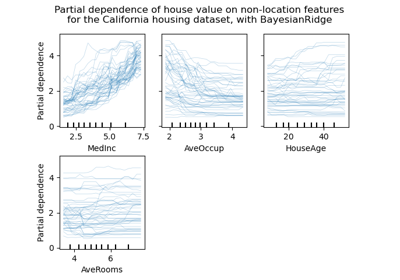Release Highlights for scikit-learn 0.24

Release Highlights for scikit-learn 0.24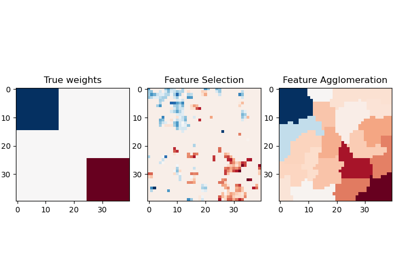Feature agglomeration vs. univariate selection

Feature agglomeration vs. univariate selection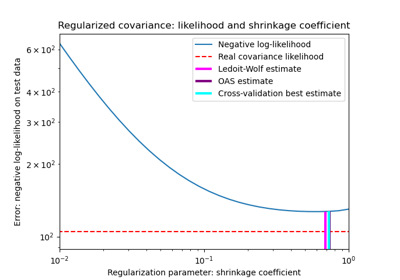Shrinkage covariance estimation: LedoitWolf vs OAS and max-likelihood

Shrinkage covariance estimation: LedoitWolf vs OAS and max-likelihood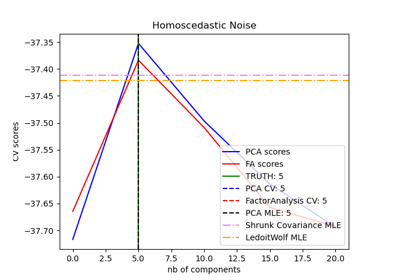Model selection with Probabilistic PCA and Factor Analysis (FA)

Model selection with Probabilistic PCA and Factor Analysis (FA)Comparing Random Forests and Histogram Gradient Boosting models

Comparing Random Forests and Histogram Gradient Boosting models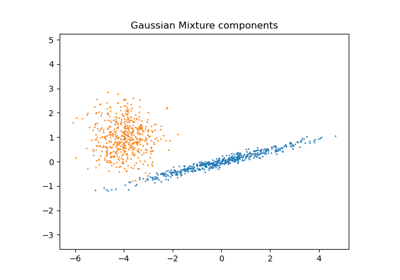Gaussian Mixture Model Selection

Gaussian Mixture Model Selection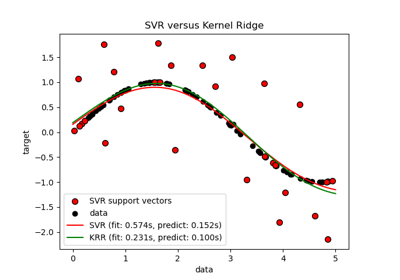Comparison of kernel ridge regression and SVR

Comparison of kernel ridge regression and SVRDisplaying Pipelines

Displaying Pipelines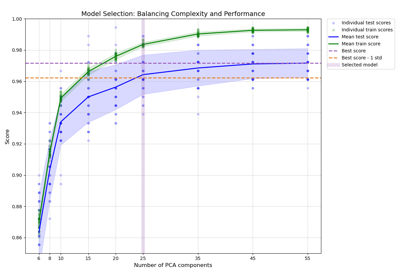Balance model complexity and cross-validated score

Balance model complexity and cross-validated scoreComparing randomized search and grid search for hyperparameter estimation

Comparing randomized search and grid search for hyperparameter estimation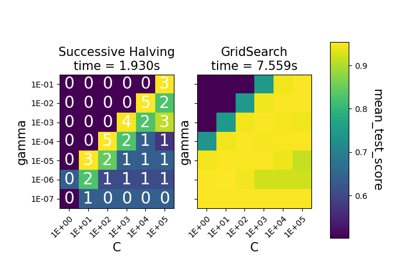Comparison between grid search and successive halving

Comparison between grid search and successive halvingCustom refit strategy of a grid search with cross-validation

Custom refit strategy of a grid search with cross-validation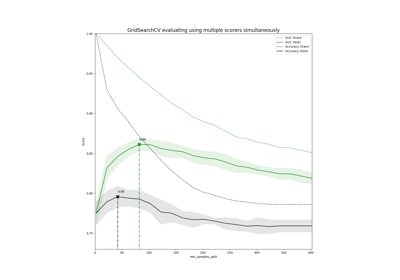Demonstration of multi-metric evaluation on cross_val_score and GridSearchCV

Demonstration of multi-metric evaluation on cross_val_score and GridSearchCV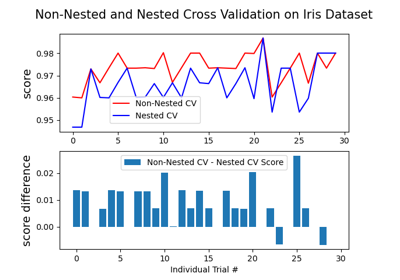Nested versus non-nested cross-validation

Nested versus non-nested cross-validationSample pipeline for text feature extraction and evaluation

Sample pipeline for text feature extraction and evaluation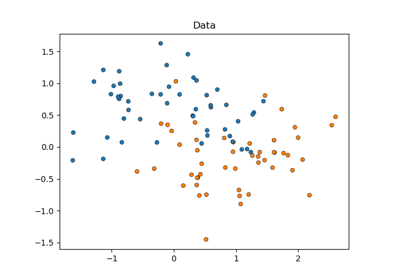Statistical comparison of models using grid search

Statistical comparison of models using grid search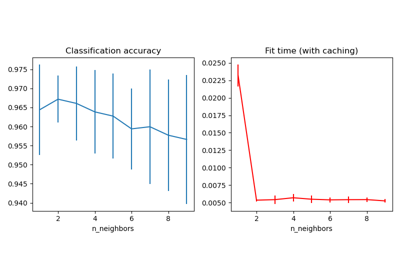Caching nearest neighbors

Caching nearest neighbors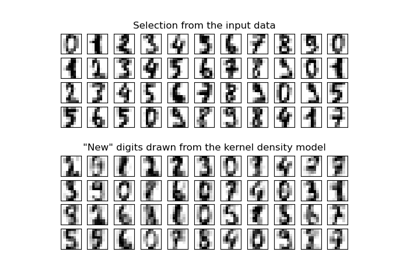Kernel Density Estimation

Kernel Density EstimationColumn Transformer with Mixed Types

Column Transformer with Mixed TypesConcatenating multiple feature extraction methods

Concatenating multiple feature extraction methods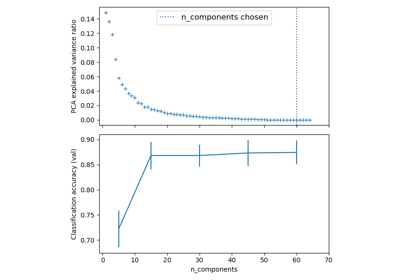Pipelining: chaining a PCA and a logistic regression

Pipelining: chaining a PCA and a logistic regression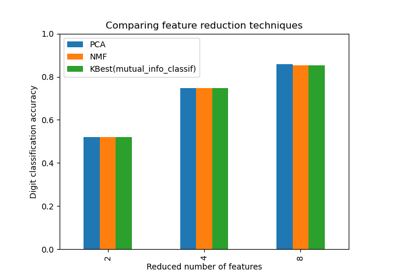Selecting dimensionality reduction with Pipeline and GridSearchCV

Selecting dimensionality reduction with Pipeline and GridSearchCV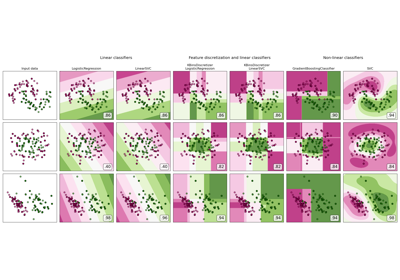Feature discretization

Feature discretization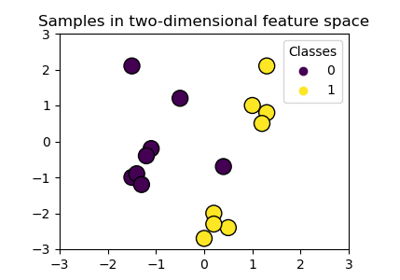Plot classification boundaries with different SVM Kernels

Plot classification boundaries with different SVM Kernels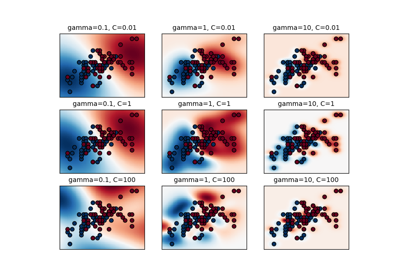RBF SVM parameters

RBF SVM parametersCross-validation on diabetes Dataset Exercise

Cross-validation on diabetes Dataset Exercise